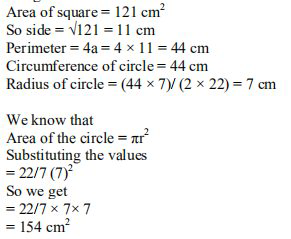# ML Aggarwal Solutions Class 9 Mathematics Solutions for Mensuration Exercise 16.3 in Chapter 16 - Mensuration

A copper wire when bent in the form of a square encloses an area of 121 \mathrm{cm}^{2} . If the same wire is bent

into the form of a circle, find the area of the circle.

It is given thatVideo transcript
"hello and welcome my dear students i am rashford the math suiter on lido and i'm here a new question it is a copper wire when bent in the form of a square encloses an area of 121 centimeter square with the same wire is bent into the form of a circle find the area of this circle so without wasting much time let's have a look at the solution so we have been given that area of square is 121 centimeters square which means that area is nothing but side square so if we apply that so side square equal to 121 gives us the side is nothing but the 11 centimeters now if we talk about the perimeter so the perimeter of square is nothing but 4 into side we can write it as 4 into s which is nothing but 4 into 11 so we get 44 centi meters which is equivalent to circumference of the circle now we know that the circumference of the circle is 2 pi r which is equivalent to 44 centimeter because the same wire has been bent to form a circle now so we'll put the values and we'll substitute we'll solve it we'll substitute the value so we get 44 into 7 divided by 2 into 22 after putting the values of pi and solving it further we get seven centimeter that means radius is nothing but the seven centi meters now if you find out the area of a circle that is equal to pi r square which is nothing but 22 by 7 into 7 square which is equals to 154 centimeter square so i hope my students got this question well do not forget to hit the subscribe button you can also share your doubts in the comment section see you next time bye"
Related Questions

Lido

Courses

Teachers

Book a Demo with us

Syllabus

Maths
CBSE
Maths
ICSE
Science
CBSE

Science
ICSE
English
CBSE
English
ICSE
Coding

Terms & Policies

Selina Question Bank

Maths
Physics
Biology

Allied Question Bank

Chemistry
Connect with us on social media!